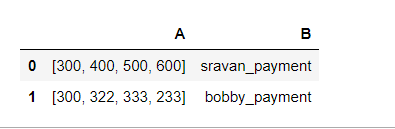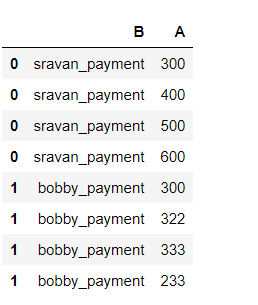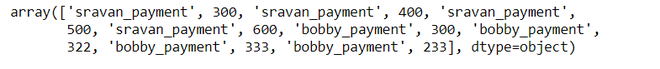# Flatten a list of DataFrames

• Last Updated : 02 Dec, 2020

In this article, we are going to see how to flatten a list of DataFrames. Flattening is defined as Converting or Changing data format to a narrow format. The advantage of the flattened list is Increases the computing speed and Good understanding of data.

Example:

Attention geek! Strengthen your foundations with the Python Programming Foundation Course and learn the basics.

To begin with, your interview preparations Enhance your Data Structures concepts with the Python DS Course. And to begin with your Machine Learning Journey, join the Machine Learning - Basic Level Course

Let consider, the data frame that contains values like payments in four months. Actually, the data is stored in a list format.Note: 0,1,2 are the indices of the records

Flattening means assigning lists separately for each author.We are going to perform flatten operations on the list using data frames.

Method 1:

Step 1: Create a simple data frame.

## Python3

 `#importing pandas module``import` `pandas as pd`` ` `#creating dataframe with 2 columns``df ``=` `pd.DataFrame(data``=``[[[ ``300``, ``400``, ``500``, ``600``], ``'sravan_payment'``], ``                        ``[[ ``300``, ``322``, ``333``, ``233``], ``'bobby_payment'``]], ``                  ``index``=``[ ``0``, ``1``], columns``=``[ ``'A'``, ``'B'``])`` ` `display(df)`

Output:Step 2: iterate each row with a specific column.

## Python3

 `flatdata ``=` `pd.DataFrame([( index, value) ``for` `( index, values)``                         ``in` `df[ ``'A'` `].iteritems() ``for` `value ``in` `values],``                             ``columns ``=` `[ ``'index'``, ``'A'``]).set_index( ``'index'` `)`` ` `df ``=` `df.drop( ``'A'``, axis ``=` `1` `).join( flatdata )``display(df)`

Output:Methods 2: Using the flatten methods.

We are going to apply the flatten function for the above code.

## Python3

 `#importing pandas module for dataframe.``import` `pandas as pd`` ` `df ``=` `pd.DataFrame(data``=``[[[ ``300``, ``400``, ``500``, ``600``], ``'sravan_payment'``], ``                        ``[[ ``300``, ``322``, ``333``, ``233``], ``'bobby_payment'``]], ``                  ``index ``=` `[ ``0``, ``1``], columns ``=` `[ ``'A'``, ``'B'``])``display(df)`

Output:## Python3

 `df.values.flatten()`

Output:My Personal Notes arrow_drop_up